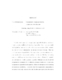## Nonparametric Quasi-likelihood in Longitudinal Data Analysis

 dc.contributor.advisor Smith, Paul J en_US dc.contributor.author Jiang, Xiaoping en_US dc.contributor.department Mathematical Statistics en_US dc.contributor.publisher Digital Repository at the University of Maryland en_US dc.contributor.publisher University of Maryland (College Park, Md.) en_US dc.date.accessioned 2005-02-02T06:41:09Z dc.date.available 2005-02-02T06:41:09Z dc.date.issued 2004-11-24 en_US dc.description.abstract This dissertation proposes a nonparametric quasi-likelihood approach to estimate regression coefficients in the class of generalized linear regression models for longitudinal data analysis, where the covariance matrices of the longitudinal data are totally unknown but are smooth functions of means. This proposed nonparametric quasi-likelihood approach is to replace the unknown covariance matrix with a nonparametric estimator in the quasi-likelihood estimating equations, which are used to estimate the regression coefficients for longitudinal data analysis. Local polynomial regression techniques are used to get the nonparametric estimator of the unknown covariance matrices in the proposed nonparametric quasi-likelihood approach. Rates of convergence of the resulting estimators are established. It is shown that the nonparametric quasi-likelihood estimator is not only consistent but also has the same asymptotic distribution as the quasi-likelihood estimator obtained with the true covariance matrix. The results from simulation studies show that the performance of the nonparametric quasi-likelihood estimator is comparable to other methods with given marginal variance functions and correctly specified correlation structures. Moreover, the results of the simulation studies show that nonparametric quasi-likelihood corrects some shortcomings of Liang and Zeger's GEE approach in longitudinal data analysis. en_US dc.format.extent 389808 bytes dc.format.mimetype application/pdf dc.identifier.uri http://hdl.handle.net/1903/2078 dc.language.iso en_US dc.subject.pqcontrolled Statistics en_US dc.subject.pquncontrolled local polynomial regression en_US dc.subject.pquncontrolled generalized estimating equations en_US dc.subject.pquncontrolled nonparametric covariance estimator en_US dc.subject.pquncontrolled regression en_US dc.subject.pquncontrolled asymptotic properties en_US dc.title Nonparametric Quasi-likelihood in Longitudinal Data Analysis en_US dc.type Dissertation en_US
##### Original bundle
Now showing 1 - 1 of 1